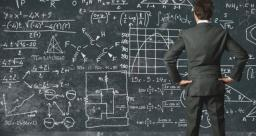# Determine 4649

Determine five solutions to the equation of two unknowns. Write as arranged pairs and draw in the graph. What does a diagram of a linear equation look like?
2x + 3y = 7

y1 =  2.3333
y2 =  1.6667
y3 =  1
y4 =  0.3333
y5 =  -0.3333

### Step-by-step explanation:Did you find an error or inaccuracy? Feel free to write us. Thank you!

Tips to related online calculators
Do you have a linear equation or system of equations and looking for its solution? Or do you have a quadratic equation?